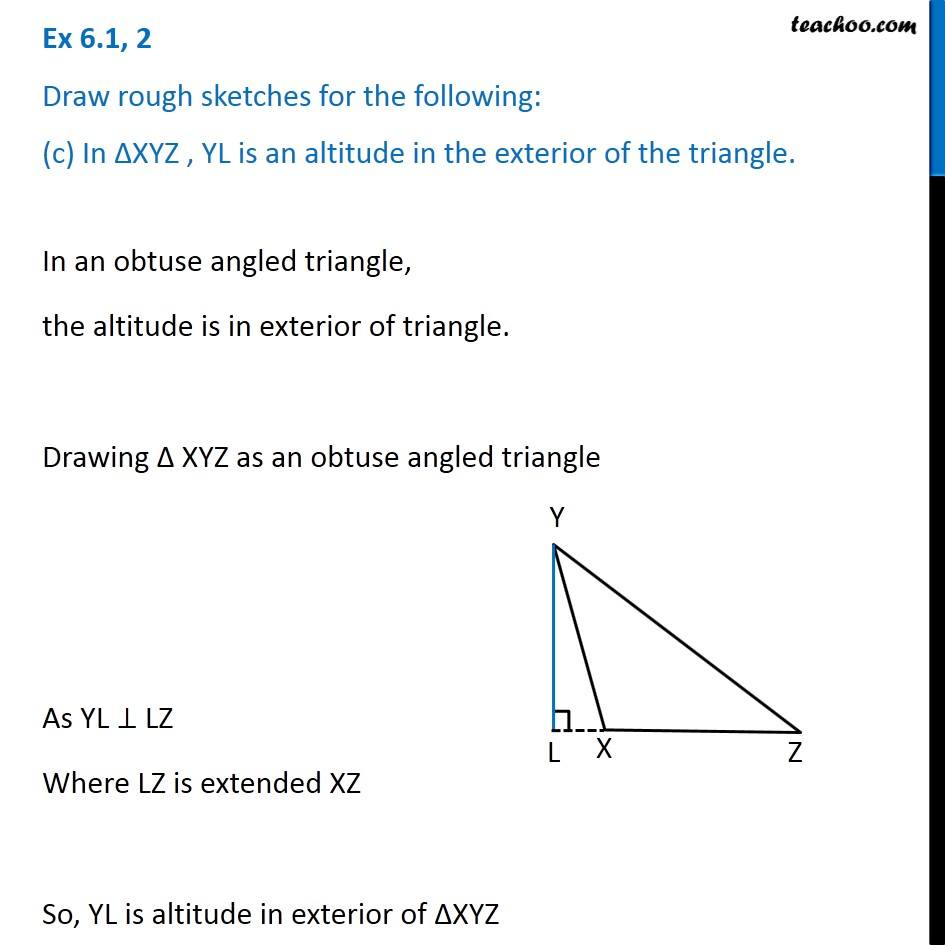1. Chapter 6 Class 7 Triangle and its Properties
2. Serial order wise
3. Ex 6.1

Transcript

Ex 6.1, 2 Draw rough sketches for the following: (c) In ∆XYZ , YL is an altitude in the exterior of the triangle. In an obtuse angled triangle, the altitude is in exterior of triangle. Drawing Δ XYZ as an obtuse angled triangle As YL ⊥ LZ Where LZ is extended XZ So, YL is altitude in exterior of ∆XYZ